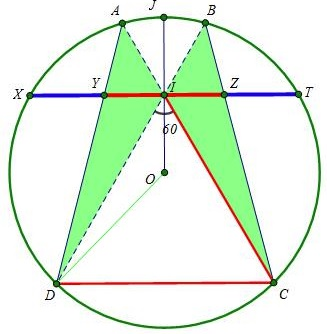# Golden Ratio in a Butterfly Astride an Equilateral Triangle

The following is taken from the post by Nguyên Dung Thanh at the CutTheKnotMath facebook page.

Let $J\;$ lie on circle $(O),\;$ with $I\;$ the midpoint of $OJ.\;$ Let $C,D\in (O)\;$ make $\Delta ICD\;$ equilateral. $IC, ID\;$ cut $(O)\;$ at $A, B,\;$ respectively. Let $XT\;$ be a chord through $I\;$ perpendicular to $OJ.\;$ $AD, BC\;$ cut $XT\;$ at $Y, Z,\;$ respectively. Then $\displaystyle\frac{YZ}{ZT}=\frac{YT}{YZ}=\varphi.$### Proof

Let $OJ=1;\;$ $ID=x;\;$ $IB=y.\;$Applying the Cosine Law in $\Delta IOD,\;$ we have

$\displaystyle OD^2=OI^2+ID^2-2OI\cdot ID\cos\angle OID,$

i.e.,

$\displaystyle 1=\frac{1}{4}+x^2-2\cdot\frac{1}{2}\cdot x\cos 30^{\circ}$

from which $\displaystyle x=\frac{\sqrt{3}}{2}\frac{\sqrt{5}+1}{2}=\frac{\sqrt{3}}{2}\varphi.\;$ Hence, $CD=ID=\sqrt{3}\varphi.$

Similarly, in $\Delta OID,$

$\displaystyle OB^2=OI^2+IB^2-2OI\cdot IB\cos\angle OIB,$

i.e.,

$\displaystyle 1=\frac{1}{4}+y^2-2\cdot\frac{1}{2}\cdot y\cos 150^{\circ}$

so that $\displaystyle y=\frac{\sqrt{3}}{2}\frac{\sqrt{5}-1}{2}=\frac{\sqrt{3}}{2}\phi.\;$ Also $BD=x+y=\frac{\sqrt{15}}{2}.$

Since $IZ\parallel CD,\;$ Applying Thales' Theorem, we have

$\displaystyle IZ=CD\cdot\frac{BI}{BD}=\frac{\sqrt{3}}{2}\varphi\cdot\frac{\sqrt{3}}{2}\phi\cdot\frac{2}{\sqrt{15}}=\frac{3}{2\sqrt{15}}$

so that $YZ=2IZ=\displaystyle\frac{3}{\sqrt{15}}.\;$ Further, $\displaystyle IT=\sqrt{OT^2-OI^2}=\frac{\sqrt{3}}{2}.\;$ Hence,

$\displaystyle ZT=IT-IZ=\frac{\sqrt{3}}{2}-\frac{3}{2\sqrt{15}}=\frac{3(\sqrt{5}-1)}{2\sqrt{15}}.\;$

Finally, we obtain

$\displaystyle \frac{YZ}{ZT}=\frac{3}{\sqrt{15}}\cdot\frac{2\sqrt{15}}{3(\sqrt{5}-1)}=\frac{\sqrt{5}+1}{2}=\varphi.$The diagram can be expanded to reveal additional occurrences of the Golden Ratio:For example,

$\displaystyle\frac{TH}{HK}=\frac{EH}{EX}=\frac{YZ}{XY}=\frac{SG}{GK}=\frac{GF}{FT}=\varphi.$### Golden Ratio